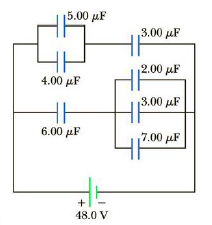Chapter 16, Problem 65AP

Chapter
Section
Textbook Problem

Find the equivalent capacitance of the group of capacitors shown in Figure P16.65.Figure P16.65

To determine
The equivalent capacitance.

Explanation

The capacitors 5.00μF and 4.00μF in parallel. Therefore,

Cp1=(5.00μF)+(4.00μF)=9.00μF

The capacitors 2.00μF , 3.00μF and 7.00μF in parallel. Therefore,

Cp2=(2.00μF)+(3.00μF)+(7.00μF)=12.0μF

The capacitors Cp1 and 3.00μF in series. Therefore,

C1=Cp1(3.00μF)Cp1+(3.00μF)=(9.00μF)(3.00μF)(9.00μF)+(3.00μF)=2

Still sussing out bartleby?

Check out a sample textbook solution.

See a sample solution

The Solution to Your Study Problems

Bartleby provides explanations to thousands of textbook problems written by our experts, many with advanced degrees!

Get Started

Find more solutions based on key concepts